# Paul McWhorter arduino lesson 9

Ah, what I basically got running here is I’ve got the code that we’ve been working with. You know for a lot of these first lessons, we’ve been working with the same schematic of the same circuit of blinking 2 LEDs we’ve just been building the code up little by little by little. If you go to my website, the address is in the description of this video. You can I get the schematic for this for this circuit. If you want to build it, and you can also get the code where you can sort of catch up to where we are, I wouldn’t recommend that you just copy this code and paste it. I would recommend that you go back and you sort of develop it yourself, because you’ve got to learn to code and the only way you learn to code is to do it. And if you just copy my paste, my code, the only thing that you will know how to do is copy and paste, and so I encourage you to go through these lessons and know how to build this code up on your own. Ok, but the thing is: is that let’s let’s zoom into one little part of this and the part that we’re going to zoom into is the part where we actually issue the command to turn the LED on or to turn the LED off and let’s come in Let’S come down inside of this loop and inside of this loop I think the last time we did it.

We were looking at while loops and so we’ve got it set up as a while loop. But as we are inside of this, while loop we’re looping it for the number of blinks that we’ve got that we’ve input from the e from the keyboard, but when we actually issue the command to the pin, we are connected on the red LED. We are connected to ten nine and a yellow LED. We are connected to pin ten, and so we are issuing commands to ten nine and pin 10. Those are set up here as variables red LED, pin, is set to nan and then yellow LED pin is set to ten and in these wires that come from the two LEDs go over to pin nine and go over to pen ten, so we’re all set up And talking to those pins again, when we’re going to talk to pins and the void setup, we’ve got to tell the Arduino what kind of communication we’re going to be doing with the pens. And so we do our pin modes. We set in pen mode red, LED pin as an output and yellow LED pin as an output, so that’s telling it pins nine in 10 we’re going to be writing voltages to now when we actually come down to write the voltages we’re using what is called a Digital write command. The argument for the digital write command is, first of all. What pin do you want to write to? We want to write to red, LED pin, and what do you want to write? We want to write it high when we write it high.

We are putting 5 volts on that pin to blanket. Then we come down to turn it off and it turn it off. We say digital write which pin red LED pin. What do you want to do? Turn it low that puts a zero on it. So we’re going apply, 5 volts, apply, zero, volts, applied 5 volt supply, zero, volts and that’s. The implication with a digital write. Digital is either an on or off it’s, either on or it’s off, there’s, not an in between with the digital write and most things and the computer will work in the digital rental on or off okay. But when we start building circuits and we start coming off of the microcontroller and coming out into the real world being oftentimes, we want something other than just 0 and 5. We want the end between voltages. We would like to maybe write 1 volt or maybe write 2 and a half volts, and in order to do that, we need to change the command and instead of doing excuse me instead of doing a digital right, we need to do an analog right and the Analog right works a lot like the digital right, so we just write analog right. We got to tell it which pin we want to write to so in this case we could still do red LED pin, but rather than high and low, we put a value in here. Okay, we put a value in here, but unfortunately it’s not as easy as applying a value between 0 and 5.

The number that we write – the right value is between 0 and 255. If we do an analog right of a right value of zero, we are applying zero volts if we do an analogue right of 255. We are writing five volts okay. So what that means is is to be able to work with this thing. We’Ve got to sort of map the world of zero to five, which is what we think in terms of zero to five volts. We got to map that on to zero to 255. Okay, and when I see the students a lot of times do is just sort of guess just get in there and put in numbers and guess, but you really really really need to be more methodical than that. So let’s just take a second and see if we can use all of this math that you have been learning for all these years in school and asking when would you ever need to know what? Well, your day is: come we’re going to use some of that math and so let’s think about this and let’s kind of think about it in terms of a line and so I’m going to come here and draw an XY axis, which hopefully you’ve been doing many Times over the last few years – and I want you to think on this axis – we’re going to have the desired voltage – this is the voltage that we would like to have and it can be between zero and five.

So let’s kind of mark that at one two, three four five yeah, I got a no it’s, not enough space there. This would be 1 volt, volts, 3, volts, 4, volts and 5 volts. Ok in order to get between zero and 5 volts. What values do we need to apply? What values do we need to write? Well, we write values between 0 and 255, and so we’ll put that on this axis. We’Ll call this one hundred we’ll call this 200 and we’ll call this 300, and so this would be about 250 and then this would be 150 and this would be 50 okay. So these are the voltages that we want, and these are the numbers that we write. Okay, what’s the first one, a are there a couple that we know zero volts. You would write what number to get zero zero. So the first number on here is the number or the point: zero zero. Okay, that’s down here is zero zero. That was an easy one. If we want five volts, what number do we write to the pin? We learn that few minutes ago 255. So if we want five, we don’t write five. We write write 255. So if we come out here to five the point that goes with, that is 255, which would be right about here. So this is the point five to fifty five okay, between any two points. We can draw a line so let’s draw it line between those two points.

Okay, and now what you can see is you can read off this line, what values that you should apply to get the voltage you want. So if you wanted a voltage of about two and a half volts let’s come up here, you would write something that would be right about there. That looks like about you know 147 or something like that, would give you the value of five. So you can use this line to figure out what values you should write to the pen to get the voltages that you want all right, but you don’t want to carry this graph around with you, the rest of your lap to try to figure out what voltage Is right, so it would be smarter to try to come up with an equation that captures the information in this line, and so let’s write an equation for the line to write an equation for the line what’s. The first thing that you need. Well, you need to get the slope. The M. The slope is equal to y2 minus y1 over x2 minus x1, okay, and so what are our two points? Five to fifty five and zero zero, so let’s put those in y2 would be 255 minus y1, which is zero over x2, which is going to be 5 minus x1, which is zero, which is going to be equal to 255 over 5 and the slope is going To be 51, ok, so now I have a slope, but I want the equation of the line.

We’Ll get the equation of the line. We can use the point slope formula because we have the slope and we have a point but it’s going to be easier. You can use whichever point you want to use the point slope formula, it’s going to be easier to use this point and so let’s use that one. You remember the point slope form of a line: it’s Y minus y1 is equal to M onto X, minus x1 B, so we’re going to have Y minus. What is y1 it’s 0 is equal to M what was m51 on 2x minus what’s X, 1, 0. Ok, and so now, what we have is y is equal to 51 times X and we can go on and sort of remind ourselves what the XampY, where the Y is going to be our right value, the value we write to the pen, the right value, the Number we write to the pin is going to be equal to 51 times the desired voltage. Okay, so the right value is 51 times the desired voltage. So if we want a voltage of 1 on the pen, what should we write? We should write 51 times the desired voltage 51 times 1. We should write 51. If we want one volt, we should write 51. If we want to volts, we should write 51 times 2, which is 102. So you see with this little equation. We can get any voltage that we want on that pen because we can calculate the right right value and go ahead and do it with the math rather than just sitting and guessing numbers until you get something that works and do it with the math okay.

So we know we’ll put this aside, but we now have the equation that allows us to get the voltage that we want on let’s see if I can get both of these things on here, all right, okay, so we’re going to start playing with this code. Now, a little bit okay, we’re going to start playing with the codes, and the first thing that we want to do is is that if we want these in between values, the first thing that we’re going to have to do is not use these digital write commands. You see how we say digital write, LED red, LED, pin high. We want to change that to an analog right, okay, so we’re going to change that to analog right and notice the case analog right with a capital W just like it was before, but now red bellied deepen hi. We still have to tell it what pen to write to, but now, instead of hi we’re going to put a number in there, and if we wanted to write one volt to that pen, one volt. What would we write here? We would. Our write value would be 51 times our desired voltage. One would be 51 that would apply one volt to it and if we applied one volt instead of five volts I’m betting, that we cannotice this to be perceivably dimmer okay, now same thing here. This still turns the thing off, but still once we start doing analog writes, I think that we should just use analog right, so we’re going to change this analog right now.

If we want to turn the pin off, we want to apply zero volts, and so what would we write value? It would be 51 times our desired voltage 51 times. Zero would be zero so to turn it off. We would do a write value of zero let’s. Do it with the with the yellow pin as well and I’m going to I’m gon na, I I’m going to say I’m going to keep my code I’m going to keep my comment good here. So here we’re going to end a log right by herb I’m going to say, we’re going to apply and log voltage to ten and then it’s that this is still good turning it off and then here what we are going to do is we are going to Apply analog voltage to e vo, yellow, LED, okay, it’s good practice disorder. I mean it’s kind of real real, easy to correct your code and to change your code and not keep your comments in line with it, and you really need to keep your comments changed as you’re changing your code, okay, so uh, and then here we are going To turn art – and this is just a perfect example of how it didn’t do that – okay and then for yellow okay, and so I think, we’ve got this. I think we’ve got this in in pretty good shape here now. I think we’ve got it in pretty good shape. Okay, so now, when I write this, I’ve got to fix these things too, so I’m going to make this analog right, so we’re going to go analog right and we want yellow, pin and we want a value of one volt and so one volt times 51.

We would write the number 51 in order to get one volt and then here we are going to analog, write a value of zero. So what we’ve done is we’ve gone into this program and we have changed our digital rights to analog rights and so let’s download. This and see what happens now, hoping it’s going to work here hanging up here, just a second. It is not happy. We make sure I got all my stuff closed out here. Okay, let’s! Try it again yeah, sometimes sometimes, if you get the orange stuff down there, you just got to try it a couple of times and sometimes it takes two or three times but but it will usually get it, and when you have that, usually you don’t have a Real good serial connection, and the best thing that you could do is to replace your replace your USB cable, which I need to do. But I need this long one because it is the only one that’ll make it all the way over here. So you’re just going to have to be patient and sometimes it just doesn’t get the okay, but it did download the code. Okay. That time it looks good. So now, let’s call up our serial, monitor and remember our program from earlier let’s. Try it again how many times you want to break blink, the red LED five. How many times do you want to blink the yellow LED five, and now it is blinking and uh.

Unfortunately, on the camera you can’t see you don’t have that much fidelity, but with my eye I can see that it’s very, very, very much dimmer. Ok, the LEDs are very, very much dimmer it’s just hard to capture that on the camera. Maybe what we need to do is maybe we need to really make it a lot lower than that, instead of one volt, how about we went like a tenth of a volt, so that would be 0.1 times 51. What if we just wrote a 5 let’s, even see if we can see that write a 5 and then down here and the other one we’re going to write a 5 by 5 as well, and so we’re analog writing a 5. What voltage are we getting if we write a value of 5 well we’re getting uh we’re getting about a tenth of a volt, because if we say the desired voltage is point one point one times 51 means we would write a number of five and let’s see If we put that down there, I’m hoping, I can get the LEDs to the point that you can actually see on the camera that they’re that they’re dimmer ok let’s, try this again, ok and most of the time. Okay, that looks like it that looks like it likes it. Ok, we’re going to blink it five times how many times you want to blink the yellow five let’s see yeah. I think you can see now that that’s really really dim.

You can, I think, even on the camera, now that you can see that that’s that that’s dimmer let’s go down. I wonder how low we can make this to where we can still see the LEDs like how small you cannot put it. Let’S let’s just let’s just for fun, see if you could see a one like if we wrote a one which would be like one fiftieth of a volt that would be really low, but let’s see if that’s enough to turn that let’s see if that’s enough to Turn the LED on and so analog write a value of 1, which would be a 50th of a bulb. So let’s see what happens there. Okay let’s call this up and then let’s say: I’m five linx and I want five blinks ooh. Can you even see it? Yeah, you can kind of see it, but you barely see it with that little voltage. If I turn the lights off that you can kind of see how to do them. That is okay. So do you see with the analog right? We can write all the numbers between zero and five volts. We can write any voltage that we want and certainly that something that’s neat to do to dim the LEDs, but you can also imagine like running servos or motors or other things, all types of different things. A lot of times you want those in between values and the way you get. Those in between values is doing an analogue right, I’d, like to just practice a little bit here, some of the stuff that we’ve learned, and so I want to go back and kind of review what we did in last week’s lesson about doing about doing input, and So this time, what I want to do is I don’t want to input the number of blinks and so I’m going to hardwire the number of blinks in here.

The number of yellow blinks I’m, going to hardwire into five is five and the number of red blinks. I am going to hardwire into five as well, and so the program, rather than getting input from the user is just going to come in and it’s going to blink the yellow, LED five times and it’s going to blink the red LED five times. Okay, but I do want to input something and what I want to input is. I want to input and let me just change this remember what three things do we have to do to get a number from a user number one. We have to prompt the user to input that number and we prompt the user doing a serial print line, and I had this set up for a different parameter, but let’s just change this to make it give us the right value that we want. Okay, so serial print line, okay, we’re going to prompt him for what number do you want to write? So what number are we going to right to that pen? Okay? Well, now? What do we do? We got ta wait for it when we prompt the user for input with a serial print line. We can do the serial print line because we’ve done the serial begin over here we can do the serial print line, prompt him for a number. What do we have to do? We have to wait and how do we wait? We wait with a while serial available is equal equals zero, do nothing, and so what this is saying is ask him for the number and then wait here on this line until he puts the number in when he puts the number in serial available.

We’Ll go to one that tells you that the number has been put in and then you come down here and read the number. But now when we read the number we don’t want to breed it in to numb red blinks. No, we want to read it in to write value that’s the value we’re going to write to that pin right that’s the value that we’re going to do the analog right with well, if we’re going to use a new variable here right value, we better declare it Up here and it’s, a number between 0 and 255, and so we’re going to make it an int we’re, going to call it right value, okay and we’re going to declare it here, declare variable or right value, okay and we’re going to have to write values because We’Re going to write a value to the red LED and then we’re also going to write a value to the yellow, LED so we’re going to have write, value, yellow and so that’s. The value that we’re going to write to the yellow, LED. Okay, keep Blair variable for all right, write value, yellow, okay, and this is declare a variable for right value red. I better put the W down here. Okay comments: it just lets us know: what’s going on Arduino, doesn’t, really read it, and so now, when we come down here, let’s read: write value red okay, and what number do you want to write to red LED? So this is the number that we’re going to write to the red LED, and so this is kind of a brightness number, and we should also probably tell the person that it needs to be between 0 and 255, just so that they know that’s.

What we’re asking for okay, how and then now for this one we’re going to input the number for the yellow LED so we’re going to use this code just kind of modified here, serial print line. And what are we going to say I’m going to say what number do you want to write to the yellow, LED? Okay, it needs to be between 0 and 255. Okay should call you put a question mark in there just be good okay, so we’re going to prompt the user after we prompt the user. What are we going to do? We’Re going to wait for his input by doing a file serial available as 0 as long as he hasn’t put the number in it’s just going to sit here looping in this little space here doing nothing until the number comes in and then he’s going to come Down here and then we need this to be right. Value yellow is equal to the number that you go, get off the serial port, so you’re going to go. Parse int you’re going to go, read an integer off the serial port, so let’s look at what we’re doing here. We’Ve just modified that old code and I hope it wasn’t too confusing, but it was easier than writing it from scratch. This time, we’ve hardwired in every time we run it the Reds going to blink the Reds going to blink 5 and the yellows going to blink 5. But the thing that we’re going to input from the user is the brightness number and the brightness number can be from 0 to 255.

If we input a number of 0, we apply 0 volts. If we input a number of 255, we apply 5 volts and so we’re scaling the voltage that we’re applying to that led from 0 to 5 volts by inputting a number from 0 to 255. Okay, the that makes sense and let’s see. I think that that should all work, but then down here now when we write the values, the analog right, we need to analog right to red, LED pin that thing that we got up here. That number, which is right, thou you read okay and then down here. We need to put in to this one right value: yellow okay, so we come up and we get the brightness value from the user for red and the brightness value from the user for yellow then down here in our analog rights, we write the red value to Red and we write the yellow value to yellow. I hope this all makes sense. Let’S run this and see if this is going to work now. Okay, I am going to have to get a new serial cable, but I do not have one so you’re in is going to have to be patient with me. Okay, there. It goes. It work that time. Okay. What number do you want to write to the red LED well, let’s, make it real real dim let’s write a 1 to it, so that would be one fiftieth of a vote.

Well, what number do you want to write to the yellow, LED a number between 0 and 255 well, let’s. Make that one really bright so let’s make that one 255, okay, and so now let’s look: what happens you see the red you can barely see. It is so dim, but the yellow is really bright. It’S turn the light off and look at that, so you see the red is at its lowest value of 50th of a volt and the yellow is at its full value of 255, which is 5 volts. You can see the yellow is blinking really really bright and the red is blinking so dim that you can’t even see it hardly okay, so let’s run this again, okay and I can actually hit the reset button to start it here again, you see the reset button. I can just hit that and it should start the program again. Okay, it says: what value do you want to write to the red LED well, let’s make the red bright this time. 255. What do you want to write to the yellow let’s? Make it one let’s, make the yellow game and see what happens? Okay, so look how bright the red is full blast in the yellow. You can barely see it. Blinking the red is full blast. The yellow. You can barely see blinking let’s try another one. Let’S make them both sort of dim, like let’s, say a tenth of a volt will a tenth of a volt.

If we wanted a tenth of a volt, it would be 0.1 times. Fifty one would be five, so let’s write five to the red and let’s write five to the yellow, so they should both be blinking, sorted in so I’m, going to write five to the red and we write five to the yellow. Okay, you see the red is kind of dim and they get kind of dim the Reds kind of dim and the yellows kind of dim okay. So what you can see here is is that, with these analog rights, we can write whatever voltage that we want between 0 and 5 volts to these Arduino pins. Okay. They’Re still, I should say that when we’re writing to these pins, even though we’re doing an analog right, we still have to do our pin modes. We still have to set those two pins up as outputs. We still have to do our pin modes, but now, when we do antelope rights instead of digital rights, we can get all the in between values between 0 and 5 volts. The thing that you just have to remember – and I see people make this mistake over and over when I’m helping students they say. Oh, I want to write 2 volts, so I do an analog write of no. You have to scale that number with the math that we did the right value that you use is 51 times the desired voltage. Okay, and if you remember that it’ll work perfectly so we’ve learned something new and powerful that now we don’t just have to turn a light on and off.

We can get a scale we can get just barely on or almost all the way on. We can control that voltage that we’re applying and, as we start, working with more sophisticated components and start working with other components besides the LED you’re just going to see how how important and how powerful that is. Okay: this has been a little bit of a tedious lesson because of all the math, but if you get confused, just go back and watch this again as far as how we went through this a equation to make this whole thing to make this whole thing work And also there’s some descriptions on the website. You can get the website from the description of this video. Okay, guys.

# Paul McWhorter arduino lesson 9 Social

(Visited 17 times, 1 visits today)

## Comment (24)

1.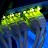aaron hancock says:

2.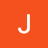Joseph Jorgensen says:

I was expecting you to ask the person to input the voltage they wanted from zero to 5 volts then adjust it in the program to the correct analog output

3.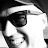Peter C says:

Great series of lessons. Much appreciate the fast upstart I’m getting. I was confused though with calling something an analog signal of specific voltage when it’s a PWM signal of a specific duty cycle. I couldn’t figure out how you were lighting an LED with such a low voltage. I understand now and keep up the great work.

4.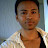boowonder888 says:

Ive had 5 years of math in total. Never used it in real life. I always say “You dont need math, you need to know basis calculus.” For all practical purposes, this statement holds true. I should have been offered BASIC instead. Cant you do the calculation with Y=aX+b? When X=0, Y=0. When X=5, Y=255……… Out of that info you can calculate the slope. Can you infer b from the graph? I think b=0 because the graph starts at point (0,0)?

5.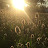Omar Omax says:

yes ,its simple math,yet easily forgotten .
you may know something but you may not know when to use it .
as i am in college trying to know when can we use what we learnt in maths,parts like integration and differentiation and graphs..etc
yes you can solve it in much more simple way
225=5
x=1
divide both equations ,tata right answer,
but still dumb and doesn’t teach you how to solve and how to think.
but in more complicated things like how to step up voltages or step down them ,,you will need a more “neat” way .and then you would be allowed to think of ways like graphs,because ,yes,you have seen that before.

6.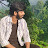bali pavankalyan says:

hello sir
as we connected(output) to the digital,
how come we get analog voltage (at output)

7.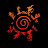Narsimha kalyan says:

What is 255 there? Is it the memory like 2^8.

8.Lorin Atzen says:

Clear… Uhum… Your… Throat… Ughum… off… Ughum camera

9.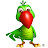Oncle Dan says:

Hi guys!
Actually, it’s not REAL analog. It is PWM (Pulse Width Modulation). 255 turns it to 5V, 100% of the time, as 0 turns it to 5V 0% of the time. Similarly, 128 turns it to 5V 50% of the time and so on.

If you really want 2,5V out of that last example, you need to put a capacitor (and a load). THEN, you’ll be able to measure your 2,5V.

An LED starts emitting light at about 2,7 Volts. That is the reason you can see it ON at a reading of 5. What you really see is full brightness about 2% of the time, then off the rest of that same time. Your eye’s perception just can’t see it blinking that fast.

Human eye’s perception is about 1/20th of a second. This is the reason a movie (film) frame rate is over that critical limit, and is actually 24 frames per second, and we see a moving object rather than a series of flickering images.

10.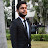Yogendra Singh says:

Why we need resistor in this when we can control voltage

11.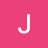Jacob Francis-Burnett says:

How does the LED that needs 2 volts to work run from 1 volt source from Arduino?

1.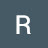Rick Louwagie says:

Pulse width modulation, they are using 0-255 to set the duty cycle. so even at 1 the 5v will turn on but for a really short time, the higher the number the longer the 5v stays on and will create a higher average voltage.. 128 will give you 50% duty cycle (2.5v average). 255 is 100% duty cycle(always on)

12.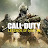the ultimate gaming channel says:

Sir you told that the voltages we apply will be assigned numbers from 0 to 255 i.e. to the lowest voltage and 255 to the highest voltage. I want to ask that what is the reason behind the voltages being numbered this way i.e. why does not the numbers go to 10k or are restricted to only 10 ? Does this have any relation with the ASCII codes ?

13.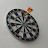krishna says:

is 0-255 an American household voltage value as in India we use 0-220, can anyone please tell me if I am thinking correctly.

1.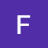Frank Fitzgerald says:

No, american household voltage is typically 0-120V. The 0-255 used in Arduino is simply a conversion

2.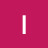Ilya shick says:

Guessing, it is binary representation of analog signal

14.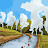Himanshu Rai says:

sir serial monitor takes value of numredblinks but it does not wait for numyellowblinks and hence yellow led is not blinking

15.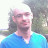David Hadley says:

I used my multimeter and spent ages trying to work out why the LEDs were so low and it was showing 1.5v. I had my GND connection in the wrong socket all the way through these tutorials! Whoops!

16.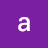acqurius 2018 says:

is there any way to control the amplitude of the PWM or square wave singal of Arduino.

17.Ilya shick says:

I guess, this formula y=51x needs to modify a little bid to better understand as-  step 1: – y/51=51/51x, step 2 – y/51=x and  >>> 0.02y=x where x is voltage on LED and y is number to enter. According to this if it is 5 v means: 5/.02 equal 255 to enter, 1 volt is 1/.02 I equal to 50, on other hand # 150 is equal 3 v,  and etc. Thanks

18.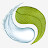Erygion says:

Thank you sir! Another lesson packed with learning and did I. The analog and pwm pins was tricky.

19.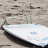Pearce Edwards says:

Anyone able to help?
Using the PWN pin with the ~ line by it. Whenever I put analogWrite(redLED, 255); with my desired amount of blinks it works fine. However if I put analogWrite(redLED, 51); it will not sequence the flashes and goes straight back to asking me how many blinks I would like. In my serial monitor it says it blinks once but I cannot see any blink on my board.

1.Pearce Edwards says:

How many blinks would you want from the red LED?
How many times would you like the Yellow LED to blink?

20.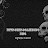NitroHiroGames bg says: